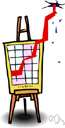# maximize

(redirected from maximizations)
Also found in: Thesaurus, Encyclopedia.

## max·i·mize

(măk′sə-mīz′)
tr.v. max·i·mized, max·i·miz·ing, max·i·miz·es
1. To increase or make as great or large as possible: "the ideal of maximizing opportunity through the equalizing of educational opportunity" (Robert J. Havighurst).
2. Mathematics To find the largest value of (a function).

max′i·mi·za′tion (-mĭ-zā′shən) n.
max′i·miz′er n.

(ˈmæksɪˌmaɪz) or

## maximise

vb
1. (tr) to make as high or great as possible; increase to a maximum
2. (Mathematics) maths to find the maximum of (a function)
ˌmaximiˈzation, ˌmaximiˈsation, ˌmaxiˈmation n
ˈmaxiˌmizer, ˈmaxiˌmiser n

## max•i•mize

(ˈmæk səˌmaɪz)

v.t. -mized, -miz•ing.
1. to increase to the greatest possible amount or degree: to maximize profits.
2. to give the highest estimate to.
3. to make fullest use of.
[1795–1805]
max`i•mi•za′tion, max`i•ma′tion, n.
max′i•miz`er, n.

## maximize

Past participle: maximized
Gerund: maximizing

Imperative
maximize
maximize
Present
I maximize
you maximize
he/she/it maximizes
we maximize
you maximize
they maximize
Preterite
I maximized
you maximized
he/she/it maximized
we maximized
you maximized
they maximized
Present Continuous
I am maximizing
you are maximizing
he/she/it is maximizing
we are maximizing
you are maximizing
they are maximizing
Present Perfect
I have maximized
you have maximized
he/she/it has maximized
we have maximized
you have maximized
they have maximized
Past Continuous
I was maximizing
you were maximizing
he/she/it was maximizing
we were maximizing
you were maximizing
they were maximizing
Past Perfect
Future
I will maximize
you will maximize
he/she/it will maximize
we will maximize
you will maximize
they will maximize
Future Perfect
I will have maximized
you will have maximized
he/she/it will have maximized
we will have maximized
you will have maximized
they will have maximized
Future Continuous
I will be maximizing
you will be maximizing
he/she/it will be maximizing
we will be maximizing
you will be maximizing
they will be maximizing
Present Perfect Continuous
I have been maximizing
you have been maximizing
he/she/it has been maximizing
we have been maximizing
you have been maximizing
they have been maximizing
Future Perfect Continuous
I will have been maximizing
you will have been maximizing
he/she/it will have been maximizing
we will have been maximizing
you will have been maximizing
they will have been maximizing
Past Perfect Continuous
Conditional
I would maximize
you would maximize
he/she/it would maximize
we would maximize
you would maximize
they would maximize
Past Conditional
I would have maximized
you would have maximized
he/she/it would have maximized
we would have maximized
you would have maximized
they would have maximized
ThesaurusAntonymsRelated WordsSynonymsLegend:
 Verb 1maximize - make as big or large as possible; "Maximize your profits!"maximiseincrease - make bigger or more; "The boss finally increased her salary"; "The university increased the number of students it admitted"minimize, minimise - make small or insignificant; "Let's minimize the risk" 2 maximize - make the most of; "He maximized his role"maximiseexploit, tap - draw from; make good use of; "we must exploit the resources we are given wisely"

## maximize

verb optimize To maximize profit, you need to maximize output.
Translations

## maximize

[ˈmæksɪmaɪz] VT [+ profits, assets, potential, opportunities] → maximizar

## maximize

[ˈmæksɪmaɪz] maximise (British) vt [+ profits, chances, support] →

## maximize

vtmaximieren; (Comput) windowmaximieren, in maximaler Standardgröße darstellen

## maximize

[ˈmæksɪˌmaɪz] vt (profits) → massimizzare; (chances) →

## maximize

vt maximizar
References in periodicals archive ?
Fujishige, Submodular Functions and Maximizations, Elsevier Science, Philadelphia, Pa, USA, 2005.
Instead of relaxing the constraints, a recent work  shows that the combinatorial cell association problem can be reformulated as a submodular maximization problem with matroid constraint and can be solved efficiently using greedy methods with low complexity.
Such a problem formulation inherits the load balancing capabilities of the utility maximization framework and discourages assigning users to a small cell with a high probability of outage.

Site: Follow: Share:
Open / Close